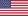# How are the Daily and Hourly Statistics calculated?

Daily statistics are calculated values for the individual day of the week over the date range selected. For example, if the date range is for 14 days, all the CGM values collected on the two Mondays would be used in the Monday calculations.
Hourly statistics are calculated values for the individual hour of the day over the date range selected. For example, if the date range is for seven days, all CGM values collected for each individual hour for each of the seven days would be used in the calculations.

LBL014183 Rev016